# PSAT Math : How to find the whole from the part

## Example Questions

### Example Question #1 : Whole And Part

Mr. Owens spent $7.50 for a dinner buffet. The amount he paid accounted for 3/4 of the money in his wallet. How much money is left in his wallet for other expenses? Possible Answers:$1.00

$2.50$6.50

$10.00$4.00

$2.50 Explanation: If$7.50 is 3/4 of the total, 7.50/3 gives us what 1/4 of his total money would be. This equals \$2.50, the remaining unspent quarter.

### Example Question #1 : Whole And Part

A certain ball that is dropped will bounce back to 3/5 of the height it was initially dropped from.  If after the 2nd bounce the ball reaches  39.96 ft, what was the initial height the ball was dropped from?

100 ft

66 ft

150 ft

135 ft

111 ft

111 ft

Explanation:

We know the height of the initial bounce, so work backwards to find the initial height.  39.96/0.6 = 66.6 = height of ball after first bounce

66.6/0.6 = 111 ft

### Example Question #3 : Whole And Part

A pitcher of water is filled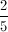of full.  An additional 27 ounces of water is added.  Now the pitcher of water is completely full.  How much water does the pitcher hold?

45

30

35

50

40

45

Explanation:

If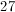ounces fills the pitcher, then it must equal the volume of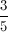of the pitcher.  Ifof a pitcher equals 27 ounces, then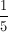of a pitcher equals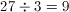ounces.  Since there arefifths in the pitcher, it must hold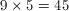ounces total.

### Example Question #82 : Arithmetic

If Mr. Jones’ math class has 8 boys and two-thirds of the class are girls, how many total students are in the class?Explanation:

If two-thirds of the class are girls, then one-third must be boys. Set up an equation comparing the number of boys to how much they represent in the entire class:

8 = (1/3) x, where x is the number in the entire class.

When we solve for x in the equation we get x = 24.

### Example Question #5 : Whole And Part

A certain bakery makes chocolate, vanilla, red velvet, and carrot cupcakes in a ratio of 2:3:5:1. If that bakery makes 63 vanilla cupcakes in one day, what is the total number of cupcakes that the bakery made that day?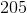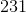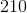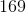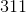Explanation:

Because the bakery makes 63 vanilla cupcakes in one day, start by dividing the 63 by the vanilla part of the ratio, which is 3.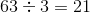. That means that the bakery made 21 times the basic set of 2 chocolate, 3 vanilla, 5 red velvet, and 1 carrot cupcakes.

Now add up the parts of your ratio: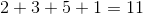. If the bakery only made 3 vanilla cupcakes, then it would have made 11 cupcakes that day. But, because the bakery made 21 times that number of vanilla cupcakes, it made 21 times the total number of cupcakes over the course of the day. To find the total number of cupcakes the bakery made that day, you multiply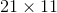to get### Example Question #6 : Whole And Part

A circle is divided into various sized slices. One slice has an interior angle of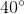and an area of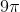. What is the circumference of the circle?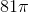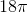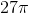Explanation:

In order to find the circumference of the cirlce, we will need to find the radius of the circle. We know that a slice with a 40-degree angle has an area of. There are 360 degrees in a circle. Therefore, the slice of the circle with an angle of 40 dgrees is one-ninth of the entire circle.

Multiplyby 9 to find the area of the circle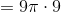The area of the circle is. Now use the formula for area to find the radius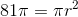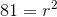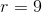The radius of the circle is 9. Use the formula for circumference to find the circumference of the circle: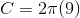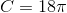The circumference of the circle is.